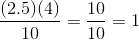## Example Questions

### Example Question #1 : How To Find The Whole From The Part With Percentage

The average cow produces 8 gallons of milk per day. Scientists have found a vitamin supplement that can safely increase a cow’s milk production by 20%. If a dairy farm consisting of 100 cows uses the supplement, how many gallons of milk will the dairy farm now produce? (Assume each cow produces the average amount of milk per day).

960 gallons

1000 gallons

800 gallons

160 gallons

960 gallons

Explanation:

First, determine the amount of milk cows will produce with the supplement: 8 + (.2 x 8)= 9.6 gallons per day. Next, determine the amount of milk 100 cows will produce when receiving the supplement: 100 x 9.6 = 960 gallons.

### Example Question #2 : How To Find The Whole From The Part With Percentage

Florida Clementines Corp sells only the best clementines. The inspector rejected 50% of January’s harvest. Unfortunately, 20% of the inspected clementines fell off the delivery truck. If the truck delivers 200 clementines, how many were harvested in January?

80

285

500

350

500

Explanation:

We first obtain how many clementines were loaded on the delivery truck from 200 delivered clementines. Then we obtain how many clementines were inspected. 200 = (1-0.2)x gives us 250 loaded onto the delivery truck. 250 = (1-0.5)x gives us 500 harvested clementines.

### Example Question #3 : How To Find The Whole From The Part With Percentage

Ed eats 3 cookies every day. If he increases the total amount of cookies he has to begin with by 50%, it would take 21 days to finish all of his cookies. How many cookies does he have to begin with?

Explanation:

We let T be the total amount of cookies and D be the number of days it takes to eat all of the cookies before increasing by 50%, and A be the number of days after increasing by 50%. With the information given, we can write the expressions, T=3D and 1.5T=3A. For the second equation, we know A=21. We plug in and get T=42.

### Example Question #4 : How To Find The Whole From The Part With Percentage

There are 8 blue balls in a bag. 40% of all the balls in the bag are blue. How many total balls are in the bag?

8

2

4

12

20

20

Explanation:

You evaluate 8/0.4 = 20.

### Example Question #1 : How To Find The Whole From The Part With Percentage

95 is 20% of what number?

500

400

475

550

475

Explanation:

multiply the part times 100 then divide by the percent.

(95 x 100) / 20 = 475

### Example Question #1 : How To Find The Whole From The Part With Percentage

If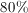of a number is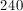, what is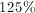of the number?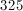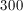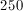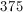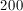Explanation:

Sinceof the number is, the number is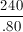which equals. Then calculateof: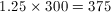### Example Question #7 : How To Find The Whole From The Part With Percentage

Gwen has a collection of 1,000 books.  The average thickness of each book is 1.25” thick.  She wants to buy enough bookshelves that all of her books will fit on it.

What is the shortest length of bookshelf she should buy, in whole feet, to fit all her books?

105 feet

120 feet

115 feet

125 feet

110 feet

105 feet

Explanation:

Length of books = 1,000 books x 1.25” per book = 1,250”

Translate to feet

1” = 12’

1250” / 12 = 104.16 feet

Round up to 105 feet to be able to fit all the books.

### Example Question #8 : How To Find The Whole From The Part With Percentage

Nathan spendsof his total homework time on Math. He spends one hour on Biology homework, which is twice the amount of time that he spends on Math. What is the total amount of time, in minutes, that Nathan spends on homework?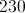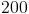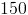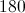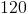Explanation:

If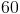minutes of Biology (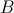) is twice as much time as that which is spent on Math (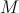), then Math must equal 30 minutes.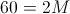, so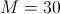.minutes is equivalent toof the total time ():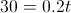Solve forby dividing both sides of the equation by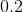.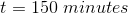### Example Question #2 : How To Find The Whole From The Part With Percentage

A bowl containsmarbles that are either red or green. The probability of selecting a red marble at random from the bowl isWhich of the following expressions represents the total number of red marbles in the bowl?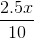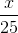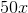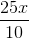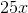Explanation:

It’s best to start with a fraction that is a part over 100. For this problem, we are looking for a fraction that shows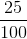.  If you go through the choices available,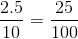. Therefore, if you plug in any value for “X” in this fraction, you will know the total number of marbles in the bowl. Here is an example:

If there are 4 marbles in the bowl, you’d expect 1 to be red. If we use this equation, we get